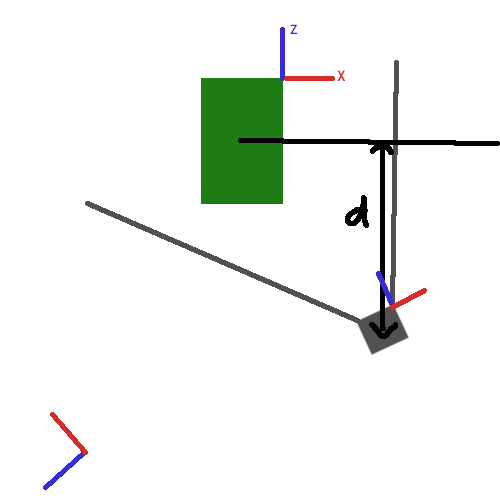# Distance with Vector3.Project

Hello, I’m trying to get distance between gameobject and camera in local space of green object. But I can’t make it work.I tried this code:

``````Vector3 dist = transform.position - green.transform.position;

Vector3 distanceZ = Vector3.Project(dist, green.transform.forward);
``````

This code return same values as before projection. I also tried .right normal but it return some weird values.

Thank anyone for help because I was trying to make this to work for few hours with no luck.

It’s not entirely clear what you actually need. The distance is just a scalar value. Unless the local space of the green object is scaled in a strange way, the distance of an object is the same in world or in global space. When you projecting a vector, the actual distance changes since, depending on the vector orienation, some part of it may be removed.

Your drawing is actually correct for what you’re doing. However your “distanceZ” is actually a world space vector that points along the normal vector you used (the forward direction of the green object). To determine that projected distance you need to use `distanceZ.magnitude` which is the distance of the camera from the imaginary plane defined by the green object and its forward vector as normal.

Note that the forward, up and right vectors of a gameobject do not represent the local space of an object. Those are just the normalized direction vector of that object’s local space, but defined in world space. It there is no scaling going on, they are the same as the basis vectors of the green object’s local space.

If you really want the distance vector between the green object and your camera in local space of the green object, you can simply use InverseTransformPoint of the green object and pass in the position of the camera. The resulting vector is the local space position of the camera as seen from the local space of the green object. So the z component is the same as the whole magnitude of your “distanceZ” vector.

``````Vector3 camLocalPos = green.transform.InverseTransformPoint(transform.position);
float dist = camLocalPos.z;
``````

Note that in this case (assuming your drawing) the z value would be negative, since your camera is “behind” the object (or in a negative z octant)

Finally note that i’ve actually assumed that the pivot of the green object is the center (since you draw the “plane line” through the center). If the pivot is where you’ve drawn your coordinate axis, you’re missing information